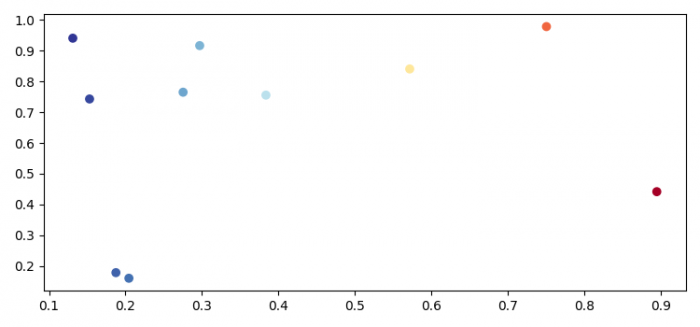# How to plot complex numbers (Argand Diagram) using Matplotlib?

MatplotlibPythonData Visualization

#### Python Data Science basics with Numpy, Pandas and Matplotlib

Most Popular

63 Lectures 6 hours

#### Data Visualization using MatPlotLib & Seaborn

11 Lectures 4 hours

#### MatPlotLib with Python

9 Lectures 2.5 hours

To plot complex numbers using matplotlib, we can make a dataset with complex numbers.

## Steps

• Set the figure size and adjust the padding between and around the subplots.
• Create random complex numbers.
• Create a figure and a set of subplots using subplots() method.
• Plot the scatter points using scatter() method.
• To display the figure, use show() method.

## Example

import numpy as np
from matplotlib import pyplot as plt
plt.rcParams["figure.figsize"] = [7.50, 3.50]
plt.rcParams["figure.autolayout"] = True
data = np.random.rand(10) + 1j*np.random.rand(10)
fig, ax = plt.subplots()
ax.scatter(data.real, data.imag, c=data.real, cmap="RdYlBu_r")
plt.show()

## Output